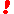# Modular Arithmetic

Modular (often also Modulo) Arithmetic is an unusually versatile tool discovered by K.F.Gauss (1777-1855) in 1801. Two numbers a and b are said to be equal or congruent modulo N iff N|(a-b), i.e. iff their difference is exactly divisible by N. Usually (and on this page) a,b, are nonnegative and N a positive integer. We write a = b (mod N).

The set of numbers congruent to a modulo N is denoted [a]N. If b ∈ [a]N then, by definition, N|(a-b) or, in other words, a and b have the same remainder of division by N. Since there are exactly N possible remainders of division by N, there are exactly N different sets [a]N. Quite often these N sets are simply identified with the corresponding remainders: N = 0, N = 1, ..., [N-1]N = N-1. Remainders are often called residues; accordingly, [a]'s are also know as the residue classes.

It's easy to see that if a = b (mod N) and c = d (mod N) then (a + c) = (b + d) (mod N). The same is true for multiplication. These allows us to introduce an algebraic structure into the set {[a]N: a = 0, 1, ..., N-1}:

By definition,

1. [a]N + [b]N = [a + b]N
2. [a]N × [b]N = [a × b]N

Subtraction is defined in an analogous manner

[a]N - [b]N = [a - b]N

and it can be verified that thus equipped set {[a]N: a = 0, 1, ..., N-1} becomes a ring with commutative addition and multiplication. Division can't be always defined. To give an obvious example,

10 × 10 = 10 × 10 = 10 × 10 = 10 × 10 = 10 × 10 = 10.

So 10/10 could not be defined uniquely. We also see that

10 × 10 = 10 × 10 = 10 × 10 = 10 × 10 = 10 × 10 = 10.

something we never had either for integer or real numbers. The situation improves for prime N's in which case division can be defined uniquely. Observe multiplication tables below for prime N. (For multiplication and division tables I have removed 0 column and row.) Every row (and column) contains all non-zero remainders mostly messed up. So every row is a permutation of the first row in the table. (This provides an easy way to construct division tables too. How?) For prime N, the set {[a]N: a=0,1,...,N-1} is promoted to a field.

### If you are reading this, your browser is not set to run Java applets. Try IE11 or Safari and declare the site https://www.cut-the-knot.org as trusted in the Java setup.What if applet does not run?

For non prime N, most rows contain zeros and repeated entries. The tables exhibit a variety of patterns. To mention a few:

1. For addition, consecutive rows result from the first one by circular rotation of entries.
2. Addition and multiplication tables are symmetric with respect to the main diagonal (it's the one that goes from the top left to the bottom right corner.)
3. Subtraction tables are not symmetric but the rows are still obtained from the first one by rotation of entries. (I subtract numbers in the leftmost column from the numbers in the topmost row.)
4.In multiplication tables, the last row is always a reverse of the first row. More generally, for base N and 1 ≤ a < N, row a is the reverse of row N - a. When N is even and hence the number of rows is odd, the middle row consists intermittently of 0 and N/2 and, in particular, is palindrom.
5.In multiplication tables modulo N, rows corresponding to numbers coprime with N contain permutations of the first row.
6.For prime (N+1), multiplication tables offer multiple and simultaneous solutions to the rook problem: on an NxN board position N rooks so that none may capture another. To solve, select a digit, replace all its occurrences with a rook, remove all other digits.
7.Under the same conditions, 1 always appears in the upper left and lower right corners and nowhere else on the main diagonal.
8. 6/5 = 4 (mod 7) or, which is the same, 7/7 = 7
9. One can use addition and subtraction tables to play the same game as with the Calendar tables.
10. For multiplication tables, this is also true, provided selected entries are multiplied instead of being added up.
11. For multiplication tables, both diagonals are palindromic: each is the same both directions.
12.If an addition table has an odd number of rows, then every remainder occurs on the main diagonal.
13. In subtraction tables with an odd number of rows, the second diagonal is a permutation of the first row.
14. In addition tables with an even number of rows, the main diagonal contains only a half of all the remainders. The remainders on the diagonal appear twice each.
15. In multiplication tables with a number of rows N where (N+1) is prime, the same is also true: the main diagonal contains only a half of all the remainders. The remainders on the diagonal appear twice each.
16. In the table of multiplication by N, rows corresponding to the numbers coprime with N consist of permutations of the first row. The reverse is always wrong.

## References

1. A. Beck, M. N. Bleicher, D. W. Crowe, Excursions into Mathematics, A K Peters, 2000
2. J. H. Conway and R. K. Guy, The Book of Numbers, Springer-Verlag, NY, 1996.
3. H. Davenport, The Higher Arithmetic, Harper&Brothers, NY
4. K. Devlin, Mathematics: The Science of Patterns, Scientific American Library, 1997
5. R. Graham, D. Knuth, O. Patashnik, Concrete Mathematics, 2nd edition, Addison-Wesley, 1994.
6. P. Hilton, D. Holton, J. Pederson, Mathematical Reflections, Springer Verlag, 1997
7. Oystein Ore, Number Theory and Its History, Dover Publications, 1976
8. S. K. Stein, Mathematics: The Man-Made Universe, 3rd edition, Dover, 2000.